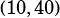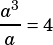In question #10 of the backsolving chapter : in the xy plane, a line containing the points (a, a^3) and (10,40) passes through the origin. Which of the following could be the value of a?

I found the explanation in the answer key to be too time-consuming if I were to solve the equation with backsolving. Can you explain how to solve this question algebraically instead?

Sure thing. Algebraically you need to key into the fact that the line passes through the origin. When a line passes through the origin, any other point on the line tells you its slope! The fact that we havetells us that the slope is 4:So, when is it true that?Choice B is, so that’s the answer.